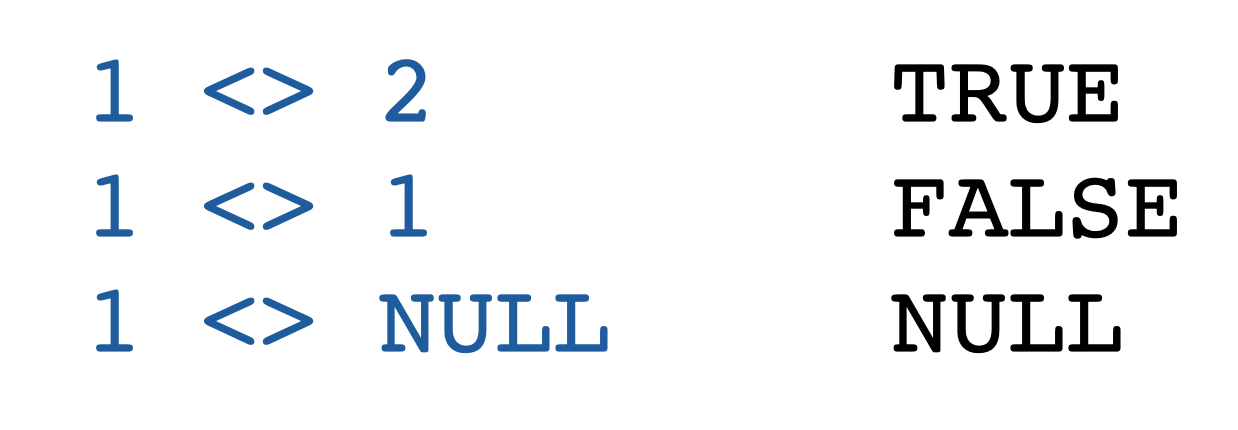Code has been added to clipboard!

# How to Use SQL Not Equal Operator

Published Sep 17, 2019
Updated Oct 3, 2019

## What Is Not Equal in SQL

The SQL Not Equal operator belongs to the group of comparison operators which means it can be used to compare expressions. In this case, an expression is a combination of symbols that has a single data value. It can be variables, constants, columns or scalar functions.

As SQL Not Equal is a binary operator, it cannot be used to compare more than two expressions at once.

## Return Values of SQL Not Equal

SQL Not Equal is a boolean operator. That means its return value can be either `True` (if one expression in SQL does not equal the other) or `False` (if the expressions are equal).

If either of the expressions has a NULL value, the SQL Not Equal will also return `Null`:## Two Types of Syntax for Not Equal

There are two ways to write the SQL Not Equal operator:

Example
``````exp <> exp
exp != exp``````

Both of them achieve the same result. However, we recommend you to use the first type of syntax, as it complies with the ISO standard, while the second one does not.